# Data Science 101 — CodingLab

Session 1

1st: import pandas as pd
2nd: Put the data into a list/dictionary
3rd:
df=pd.DataFrame(data,column=[‘….’, ‘….’, ‘….’])
df

*note: Dictionary key(column name)-value(data) pair

import pandas as pd
df

3. Data Processing

Extracting Row from DataFrame:

df = pd.DataFrame(data,columns = [‘name’,’age’,’salary’])
df.loc → ‘0’ refers to the indexing

Extracting Column from DataFrame:

df = pd.DataFrame(data,columns = [‘name’,’age’,’salary’])
df[‘name’] → means the ‘name’ column

Grouping & Aggregation:

class_score = df.groupby(‘Class’).agg({‘Score’: [‘mean’, ‘min’, ‘max’]})
class_score

Grouping by Multiple Columns:

class_score = df.groupby([‘Class’,’Age’]).agg({‘Score’: [‘mean’, ‘min’, ‘max’]})
class_score

Filtering: Select Rows Based on Value of Column:

year_2002 = df[(df[‘year’] == 2002)]
year_2002

To get the original dataframe, just type: print(df)

Select Rows Whose Column Value Does NOT Equal a Specific Value:

year_not_2002 = df[ (df[‘year’] != 2002)]
year_not_2002

Those that are not year 2002 will be displayed.

There is two ways df[‘year’] and df.year

df[‘…’ ] is a better method if the header is long with spaces. However, df.year will be a faster method.

Select Not Null:

df_not_null = df[df.year.notnull()]
df_not_null

Select rows based on a list:

years = [2002,2008]
selection = df[df.year.isin(years)]
selection

Select Rows Based on Values Not in List:

The opposite is called negate ~

years = [2002,2008]
selection = df[~df.year.isin(years)]
selection

Select Rows Using Multiple Conditions:

Want to have more than one conditions. Use ‘and’ → &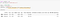Specify two conditions; year → 2002,2008 and country → Singapore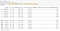Two conditions; year → range 2004 to 2009(ie. between 2004 and 2008) and country other than Singapore

Note for using print():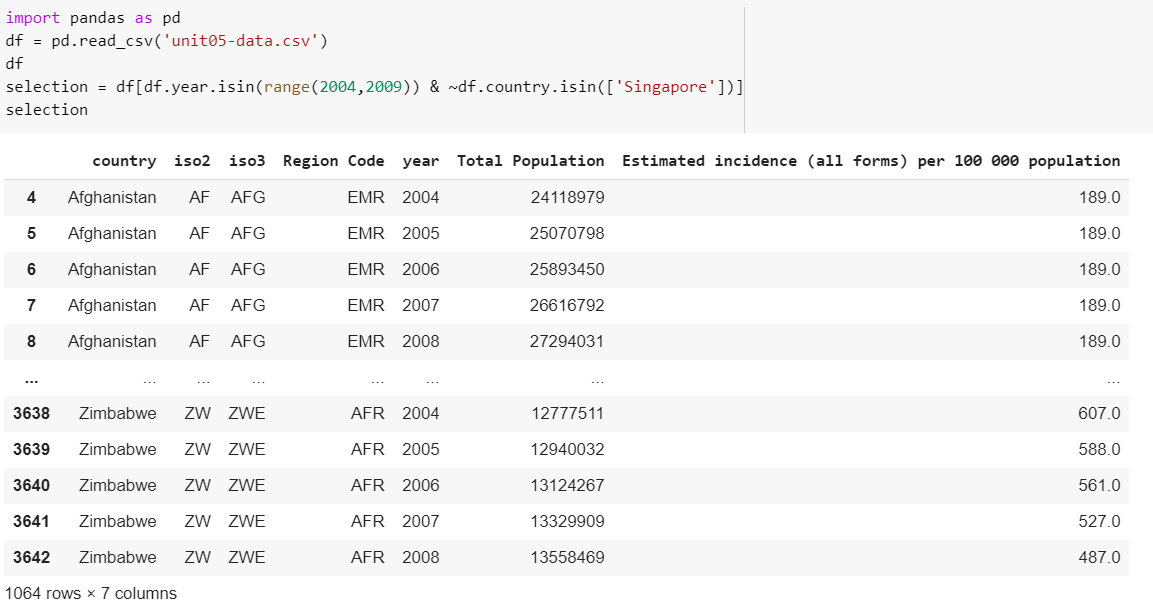(left) with the print(), both df and selection will be printed. (right) without print(), only the last variable will be printed, in this case, selection.

Concatenate DataFrames by Rows:

Concatenate by Column:

axis=1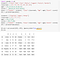the new data frame will be join as new column, which is not logical in our example

The ‘NaN’ means empty/null. Because df2 only has 3 rows of data, so the rest were displayed as ‘NaN’.

Merge DataFrames:

Pivoting in Pandas:

aggfunc → aggregation function by default will calculate the mean/average

index → Refers to the row

values → what data you want to display on the data frame.

fill_value → will display all ‘NaN’ with ‘0’ instead.

Set Missing Values To 0: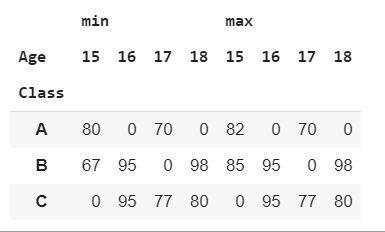Calculate minimum and maximum score and set missing values to ‘0’

Output DataFrame to csv:

.to_csv

r → raw data means a full stop will be read as full stop

4. Data Cleaning

Missing Data: Either remove data or use imputation

i) remove data

By default dropna() will only remove row.

dropna(axis=1) will remove column.

ii) Data Imputation

Removing data may be convenient, but it may delete some important information on other columns.

Imputation means editing the values by some other values.

.fillna(0)

df[‘Age’].mean()==df.Age.mean()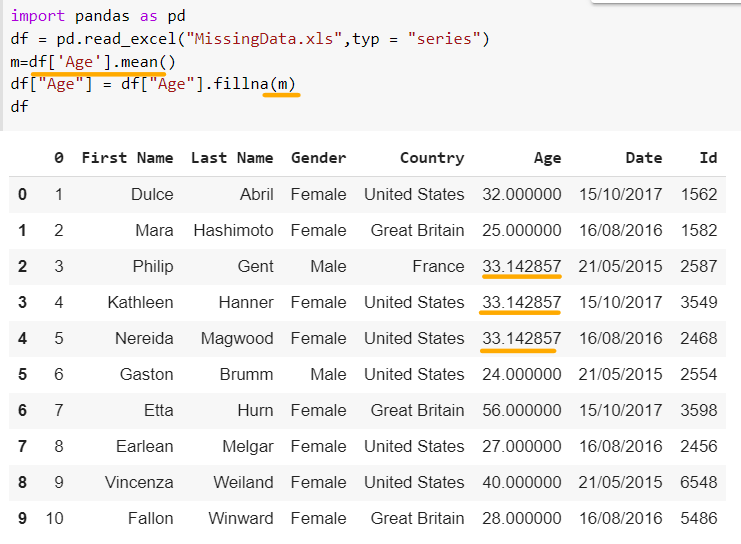Assign new variable ‘m’ and replace ‘NaN’ with the mean age.

Plotting Histogram:

Quantitative(t-test,z-test,chi-square, Anova test), Graphical

Plotting Boxplot:

px.box( df, x= ,y= )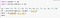‘Y1’ is just a name and it can be changed to any naming.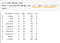You can change the ‘Age’ to ‘Score’ or other things

Plotting Bar Graphs:

Bar graph has spaces, while histogram is stick together.

Usually used for data with different category.

px.bar( df, x= , y= )

Plotting Pie-Chart:

px.pie(df, values= , names=)

values → data values

names → axis

Session 2

Descriptive statistics using data collected from a population through numerical calculations or graphs or tables.

Inferential statistics using sample data taken from a population to make inferences/predictions.

Confidence interval tells you the chances of meeting a certain range/criteria. eg.95%CI means 5% chance will not fall within the range.

Hypothesis Testing(z-test)

The higher the confidence level, the higher the z-score. (eg. You are confident that the mean height of people will fall within the range 1.4m to 1.8m, which is highly probable. But also mean higher margin of error thus higher z-score.)

one-tailed test, two-tailed test

level of significance(rejection level for two-tailed test)==alpha level

Z-test compare the mean of one sample/hypothesis
T-test compare the mean of two sample/hypothesis
ANOVA Test(Analysis of Variance) compare the mean of more than two sample/hypothesis
Chi-Square test compare categorical variables.

statistically significant means you are able to get the same result when you repeat the test on another sample. (It must be able to produce the same results when tested over and over again)

The more sample size (ie.30 samples and above), the more likely you will get a normal distribution.

Outliers normally we will keep them in your data unless you are really sure the outlier is due to errors(eg. recorded wrongly)

To determine data is normal:

1. central limit theorem (>30 sample size)
2. Chi-square test for normality
3. plot a graph and see if the shape is a normal distribution

IQR allows you to determine/identify your outlier.

Session 3

Scipy — https://docs.scipy.org/doc/

When do we use z-test?
Population standard deviation is known
Data should be normally distributed(ie. sample size >30 → central limit theorem)

statmodels gives you the pval and zset for the entire sample.
scipy.stats give you each indiviual value in the sample.

When do we use t-test?

When population variance/SD is unknown.
When determining the difference between the mean of 2 groups of sample.
Data should be normally distributed
For small sample size <30

Use Anova test when comparing data from more than two groups

Anova test == F-test

F-value = 7.1210194…(its same as the T-score, Z-score)

Chi-square is for data with categories.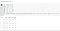creating the dataframe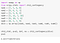pval>0.05, therefore, we do not reject null hypothesis. We conclude that there is not enough evidence to suggest the dice is not fair(this does not equate to being a fair dice).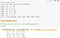If we print everything, it has 4 values. Refer to the image below for legend.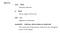https://docs.scipy.org/doc/scipy/reference/generated/scipy.stats.chi2_contingency.html

p value is the probability of obtaining the test results are at least at the tails of the bell curve. Lower p value means to reject null hypothesis(ie <0.05)

rvalue → correlation of your values

matplotlib → draw graphs